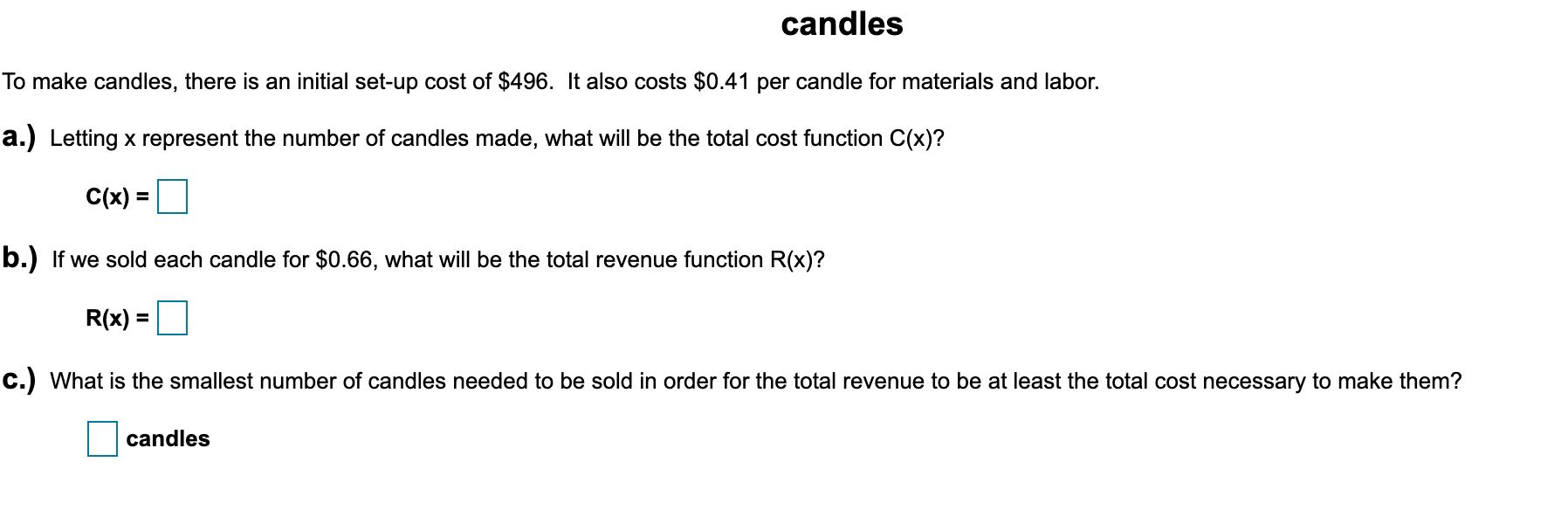# candles To make candles, there is an initial set-up cost of $496. It also costs$0.41 per candle for materials and labor. a.) Letting x represent the number of candles made, what will be the total cost function C(x)? C(x) = %3D b.) If we sold each candle for $0.66, what will be the total revenue function R(x)? R(x) = c.) What is the smallest number of candles needed to be sold in order for the total revenue to be at least the total cost necessary to make them? candles Questionhelp_outlineImage Transcriptionclosecandles To make candles, there is an initial set-up cost of$496. It also costs $0.41 per candle for materials and labor. a.) Letting x represent the number of candles made, what will be the total cost function C(x)? C(x) = %3D b.) If we sold each candle for$0.66, what will be the total revenue function R(x)? R(x) = c.) What is the smallest number of candles needed to be sold in order for the total revenue to be at least the total cost necessary to make them? candles fullscreen

### Want to see this answer and more?

Experts are waiting 24/7 to provide step-by-step solutions in as fast as 30 minutes!*

*Response times may vary by subject and question complexity. Median response time is 34 minutes for paid subscribers and may be longer for promotional offers.
Tagged in
Math
Algebra

### Applications of Mathematics